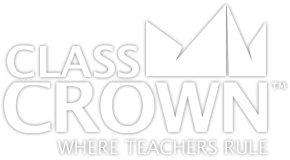## 5th Grade Math Worksheets: Pack 1

Skills included in this pack:
• Solving with parentheses (easier)
• Solving with parentheses
• Rewriting number sentences (with parentheses)
• Graphing using given rules
• Finding digit values with decimals
• Examining digit place values
• Decimal equation patterns
• Decimal equation patterns
• Multiplying and dividing patterns of ten
• Solving decimal word problems with power of ten
• Understanding multiplying decimals
• Dividing multiples of 10
• Understanding multiplying by 10s and 100s
• Understanding multiplying by 10s, 100s, and 1000s
• Converting decimals from word to numeric form
• Converting expanded notation to numeric form with decimals
• Converting expanded notation to numeric form with decimals
• Converting numeric form to expanded notation with decimals
• Converting numeric form to word form with decimals
• Comparing decimals (thousandths)
• Ordering decimals
• Rounding decimals
• Multiplying 3-digit by 2-digit
• Multiplying 3-digit by 2-digit
• Multiplying 4-digit by 2-digit
• Multiplying 4-digit by 2-digit
• Multiplying multi-digit whole number word problems
• Dividing 4-digit by 2-digit with remainder
• Dividing 3-digit by 2-digit with remainder
• Dividing 4-digit by 2-digit with remainder
+ See More
Free Sample Pages Available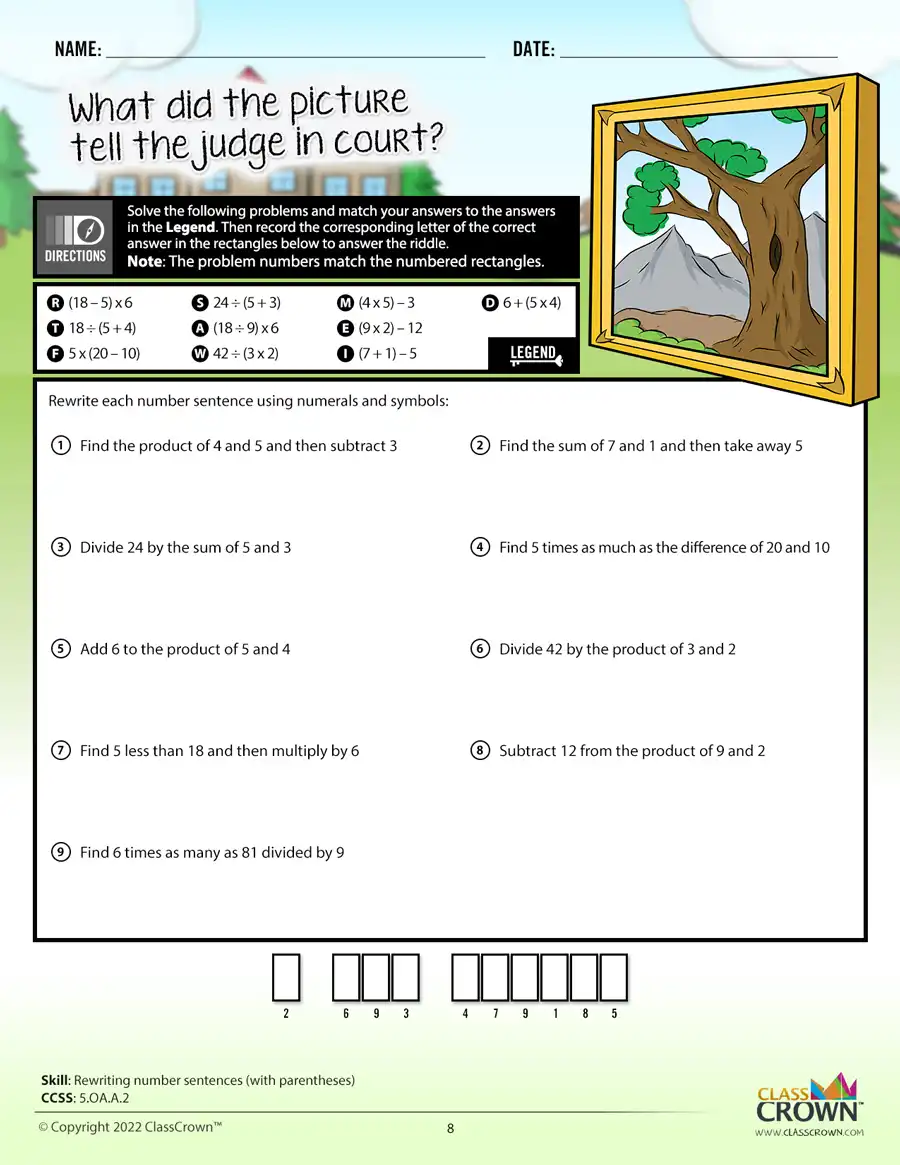## 5th Grade Math Worksheets: Pack 2

Skills included in this pack:
• Adding and subtracting decimals (horizontal)
• Adding and subtracting decimals word problems
• Adding and subtracting decimals with number lines
• Dividing decimals (decimals in dividend and divisor)
• Dividing decimals (decimals in dividend and divisor)
• Multiplying decimals (vertical)
• Multiplying decimals (horizontal)
• Multiplying decimals visually
• Multiplying decimals visually
• Adding and subtracting fractions with unlike denominators, visual
• Adding and subtracting fractions with unlike denominators
• Adding and subtracting mixed numbers with unlike denominators, no regrouping
• Adding and subtracting mixed numbers with unlike denominators, with regrouping
• Adding and subtracting fractions with unlike denominators, word problems
• Adding and subtracting fractions with unlike denominators and word problems review
• Fractions as division problems
• Examining fraction value
• Fractions as division problems, word problems
• Multiplying fractions by whole numbers with number lines
• Multiplying fractions, different denominators
• Multiplying mixed numbers
• Multiplying fractions, visual
• Finding and comparing products
• Estimating multiplication of fractions
• Multiplying fractions, word problems
• Multiplying fractions with cross-canceling
• Dividing fractions by whole numbers
• Dividing whole numbers by fractions
• Dividing whole numbers by fractions, visual
+ See More
Free Sample Pages Available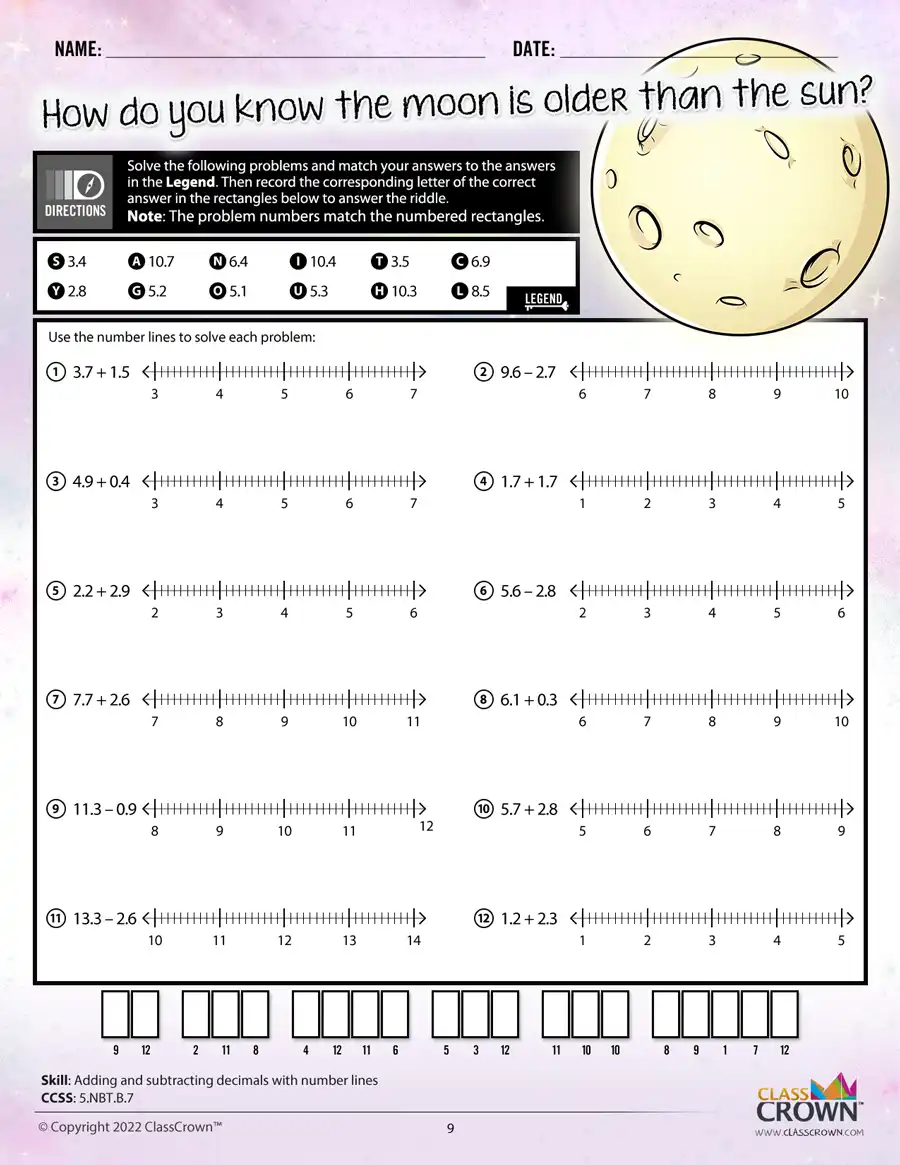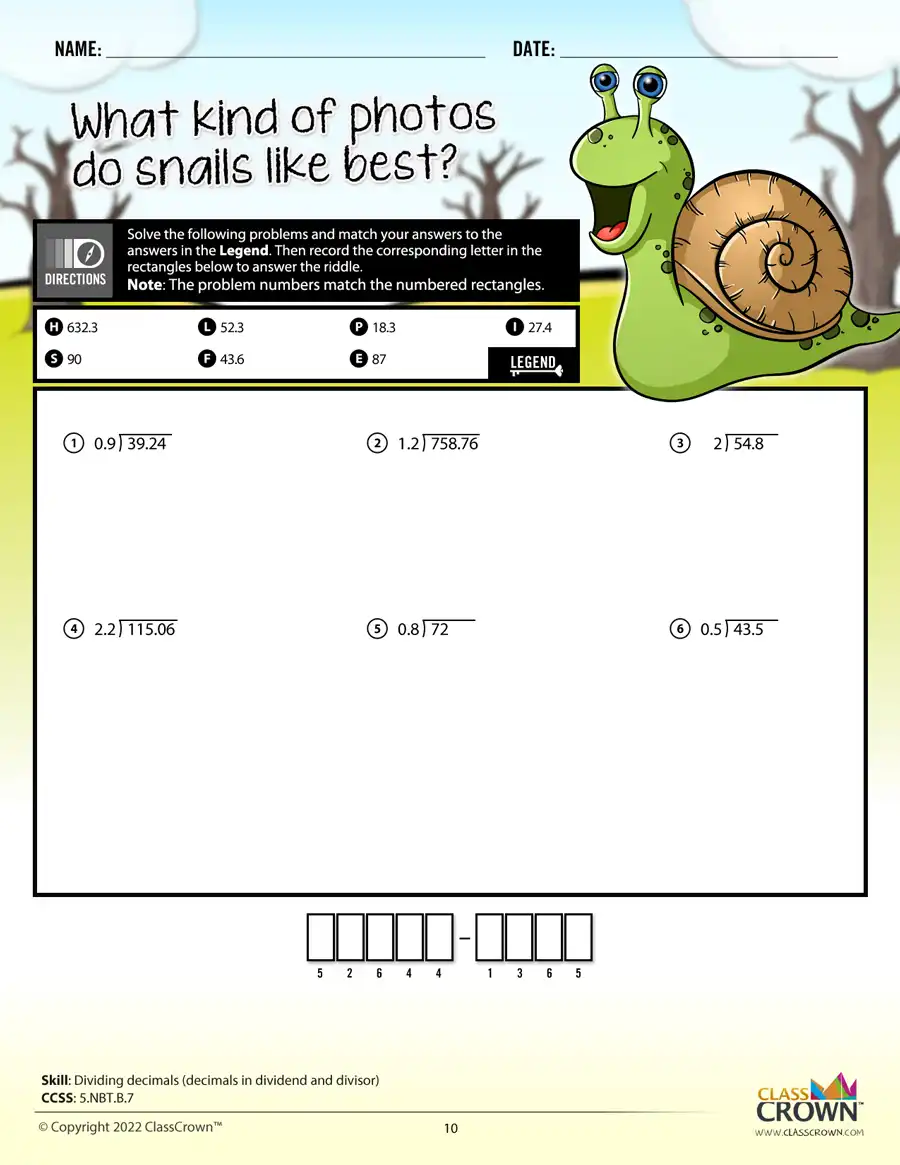## Pre-algebra Worksheets: Pack 1

Skills included in this pack:
• Subtracting integers
• Multiplying integers
• Dividing integers
• Adding, subtracting, multiplying, and dividing integers
• Simplifying using order of operations
• Writing variable expressions
• Evaluating variable expressions
• Writing equations to represent word problems
• Completing function tables
• Solving 1-step equations by adding/subtracting
• Solving 1-step equations by multiplying/dividing
• Solving 2-step equations (includes integers)
• Writing linear functions
• Graphing linear functions
• Evaluating functions
• Writing multiplication expressions using exponents
• Evaluating exponents
• Multiplying powers
• Dividing powers
• Using scientific notation
+ See More
Free Sample Pages Available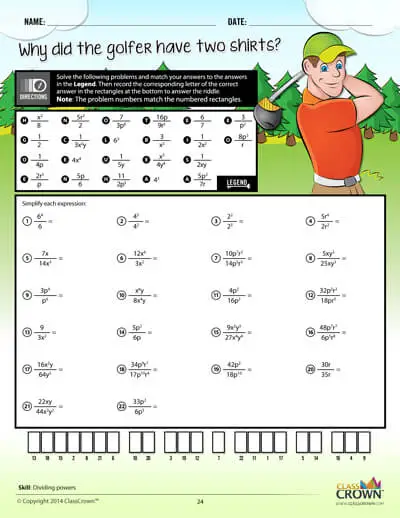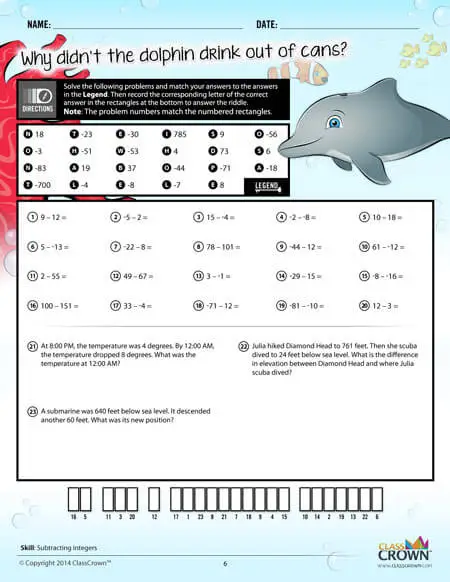## Ratios & Percents Pack 1

Skills included in this pack:
• Determining ratios
• Ratio word problems
• Equivalent ratios
• Proportions
• Proportions word problems
• Similar figures and proportions
• Representing percentages graphically
• Converting between fractions, decimals, and percents
• Converting fractions to percents
• Converting percents to fractions
• Converting between decimals and percents
• Estimating percents using benchmark fractions
• Percent of a number
• Finding percents – numbers of numbers
• Finding a number when a percent of it is known
• Discount and sale prices
• Calculating sales tax
• Percents word problems
• Percents problem solving, mixed
• Ratios and percents, mixed applications
+ See More
Free Sample Pages Available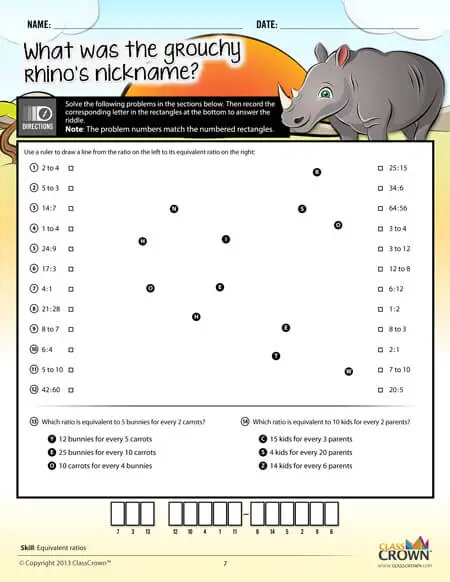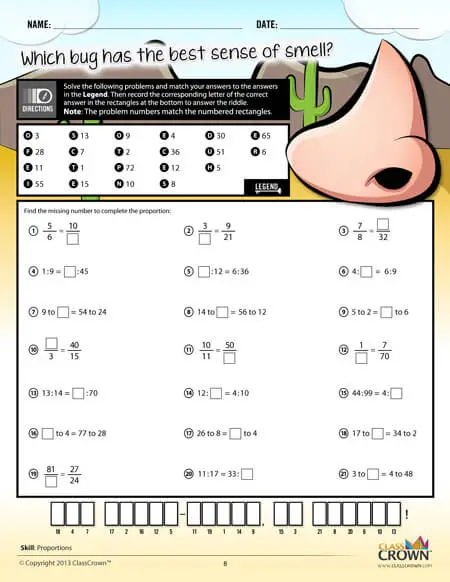## Geometry Worksheets: Pack 2

Skills included in this pack:
• Identifying parts of a circle
• Finding the perimeter of figures
• Finding the area of squares and rectangles
• Finding the area of triangles
• Finding the area of complex figures with right angles
• Finding the area between rectangles
• Finding the perimeter and area of rectangles, triangles, and complex figures with right angles
• Finding the volume of rectangular prisms
• Finding the radius and diameter of a circle
• Finding the area of a circle
• Finding the circumference and area of circles
• Finding the area of parallelograms and trapezoids
• Finding the circumference of a circle
• Finding surface area of cylinders, rectangular prisms, and right square pyramids
• Finding surface area with word problems
• Finding the volume and surface area of rectangular prisms
• Finding the volume and surface area of cylinders
+ See More
Free Sample Pages Available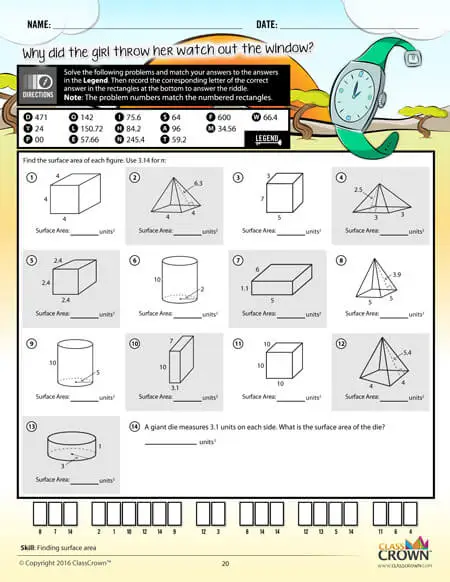## Geometry Worksheets: Pack 1

Skills included in this pack:
• Identifying planar figures
• Identifying a shape/figure when described
• Identifying types of triangles as scalene, isosceles, or equilateral
• Identifying similar and congruent figures
• Identifying nets
• Identifying acute, obtuse, and right angles
• Identifying acute, obtuse, and right triangles
• Using a protractor to find the measure of an angle
• Estimating angle measures
• Finding missing angles using properties of adjacent angles
• Identifying lines of symmetry
• Identifying lines, line segments, and rays
• Identifying parallel, perpendicular, and intersecting lines
• Identifying rotations, reflections, and translations
• Finding unknown angles in triangles and quadrilaterals
• Identifying complementary and supplementary angles
• Identifying number of sides, vertices, edges, and faces of 3-dimensional figures
+ See More
Free Sample Pages Available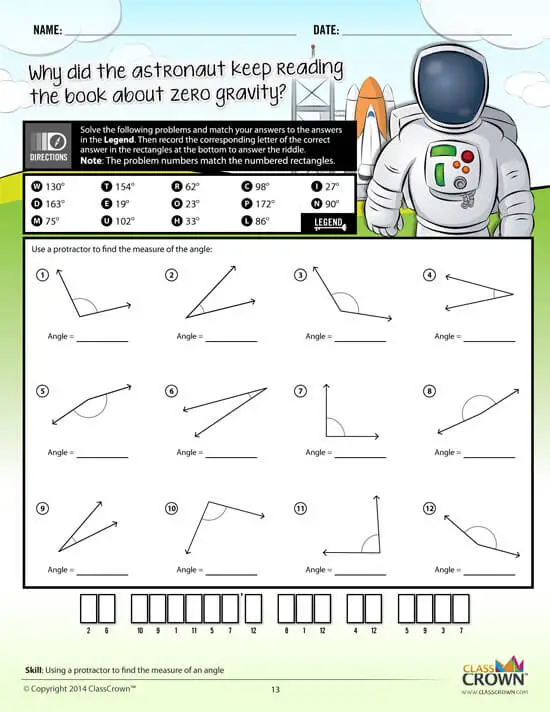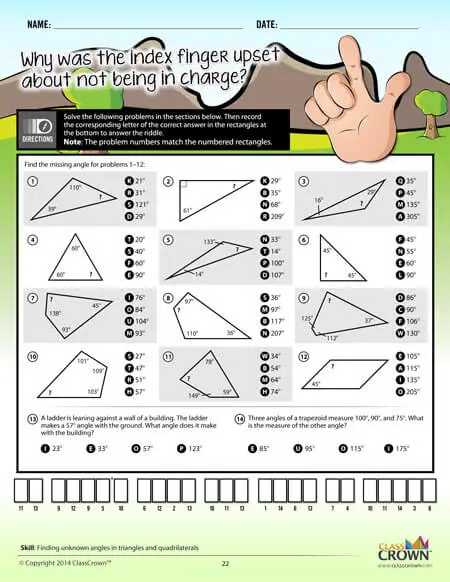## Decimals Worksheets: Pack 1

Skills included in this pack:
• Naming place value to the thousandths place
• Decimals expressed as pictures
• Decimals expressed in words
• Rounding decimals to the hundredths place
• Identifying decimals on a number line
• Comparing decimals
• Decimals expressed as fractions
• Comparing decimals with fractions
• Subtracting decimals
• Adding and subtracting decimals word problems
• Multiplying decimals by powers of 10
• Multiplying decimals by one-digit whole numbers
• Multiplying decimals by two-digit whole numbers
• Representing multiplication of decimals visually
• Multiplying decimals
• Dividing decimals by powers of 10
• Dividing decimals by whole numbers
• Dividing with decimals in the divisor
• Division: adding a decimal and zeros to the dividend
+ See More
Free Sample Pages Available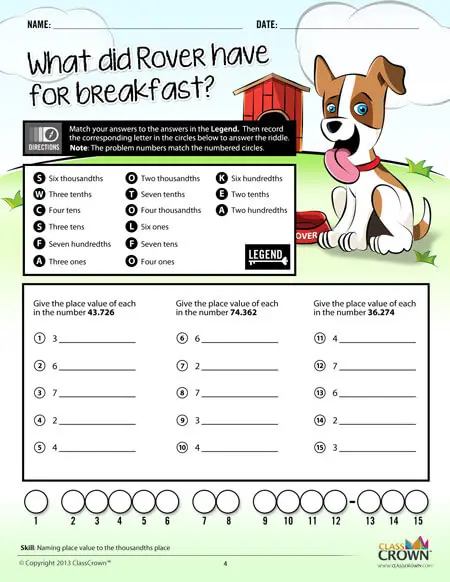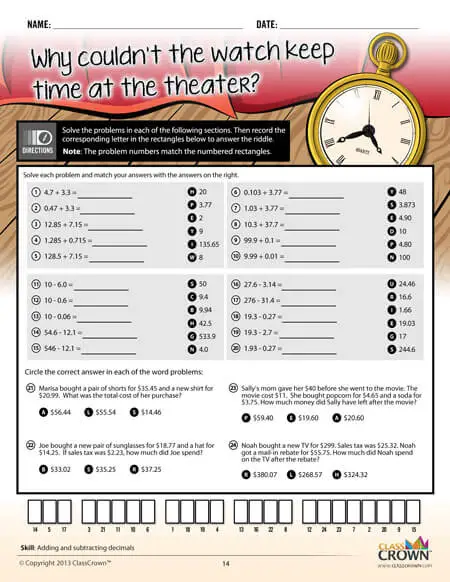## Fractions Pack 1: Concepts

Skills included in this pack:
• Identifying prime and composite numbers
• Prime factorization
• Prime factorization with exponents
• Using divisibility rules
• Using divisibility rules in word problems
• Finding greatest common factor
• Identifying equivalent fractions
• Simplifying fractions
• Converting improper fractions into mixed numbers
• Converting mixed numbers into improper fractions
• Identifying fractions on a number line
• Comparing fractions
• Comparing decimals and fractions
• Converting between decimals and fractions
+ See More
Free Sample Pages Available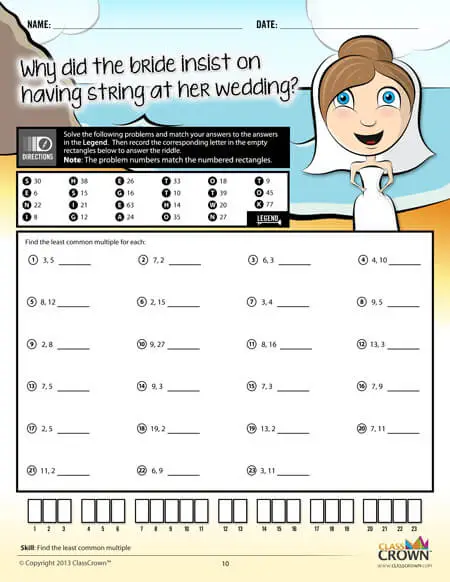## Fractions Pack 2: Adding & Subtracting

Skills included in this pack:
• Adding fractions with like denominators
• Adding mixed numbers with like denominators, no regrouping
• Adding fractions with unlike denominators
• Subtracting fractions with like denominators
• Subtracting mixed numbers with like denominators
• Subtracting fractions with unlike denominators
• Adding mixed numbers with unlike denominators
• Subtracting mixed numbers from whole numbers
• Subtracting fractions with unlike denominators and with regrouping
• Adding and subtracting fractions word problems
• Adding and subtracting mixed numbers word problems
• Adding/subtracting fractions and mixed numbers
• Adding three or more fractions with unlike denominators
• Inequalities with adding and subtracting fractions
• Estimating sums of fractions
+ See More
Free Sample Pages Available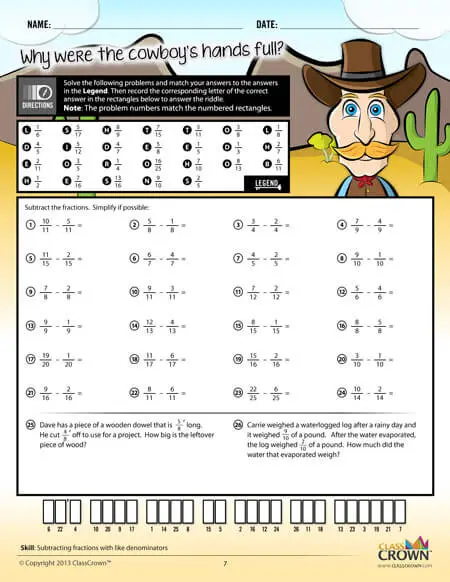## Fractions Pack 3: Multiplying & Dividing

Skills included in this pack:
• Multiplying whole numbers by fractions
• Multiplying whole number by fractions, word problems
• Representing multiplication of fractions visually
• Multiplying two fractions
• Multiplying two fractions, word problems
• Multiplying three fractions
• Multiplying a mixed number by a whole number
• Multiplying mixed numbers and fractions
• Multiplying two mixed numbers
• Dividing whole numbers by fractions
• Dividing fractions by whole numbers
• Dividing two fractions
• Dividing fractions and mixed numbers
• Dividing two mixed numbers
• Multiplying and dividing fractions and mixed numbers, word problems
+ See More
Free Sample Pages Available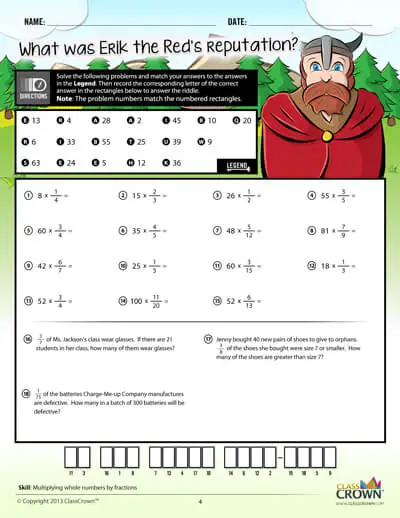Self Checking

Our math worksheets introduce a puzzle aspect to math, giving students immediate feedback as to whether or not they are solving problems correctly. If the answer to the riddle isn't spelled correctly, the student knows which problems he's made an error on.Fun Puzzle AspectImmediate Feedback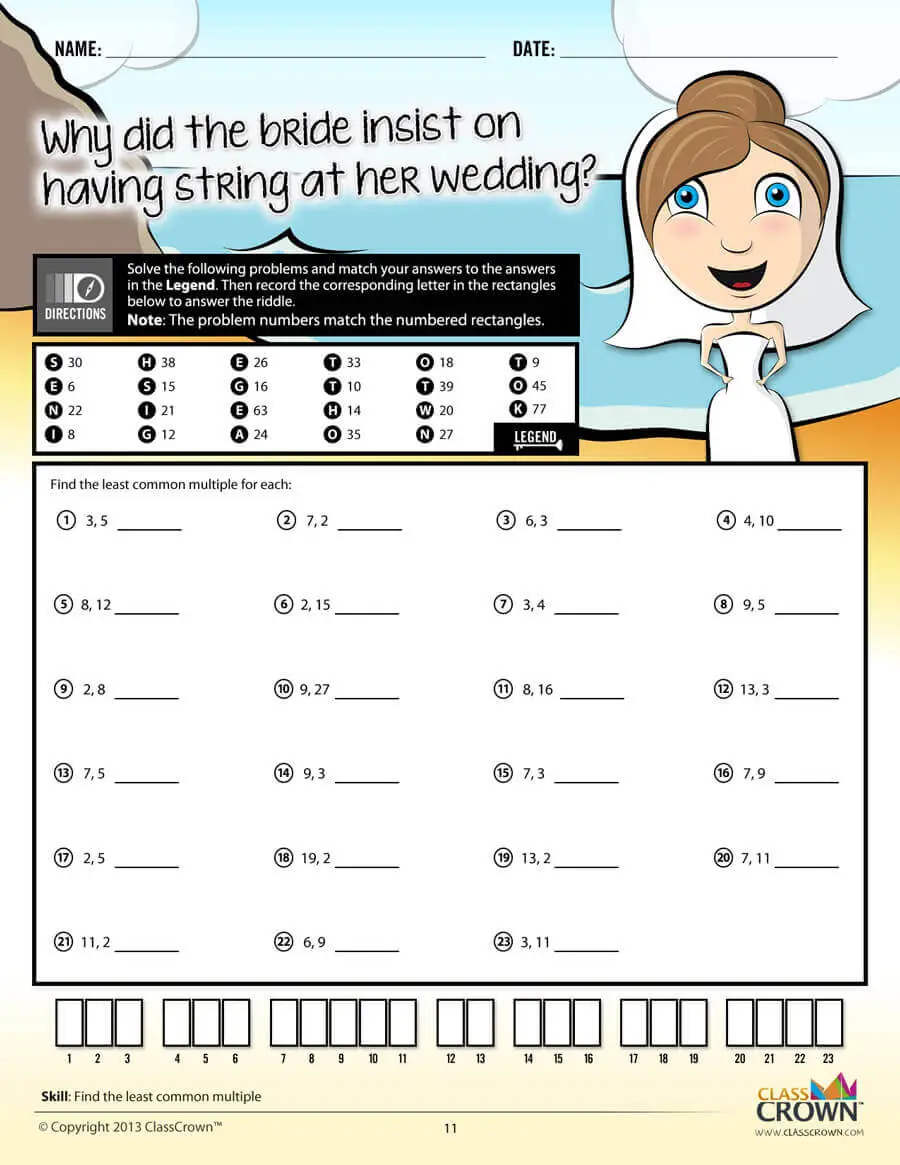Problem Solving Motivation

Each math worksheet contains a riddle that the student solves by completeing all the problems on the worksheet. This keeps kids motivated to complete each problem so that they can find the answer to the riddle.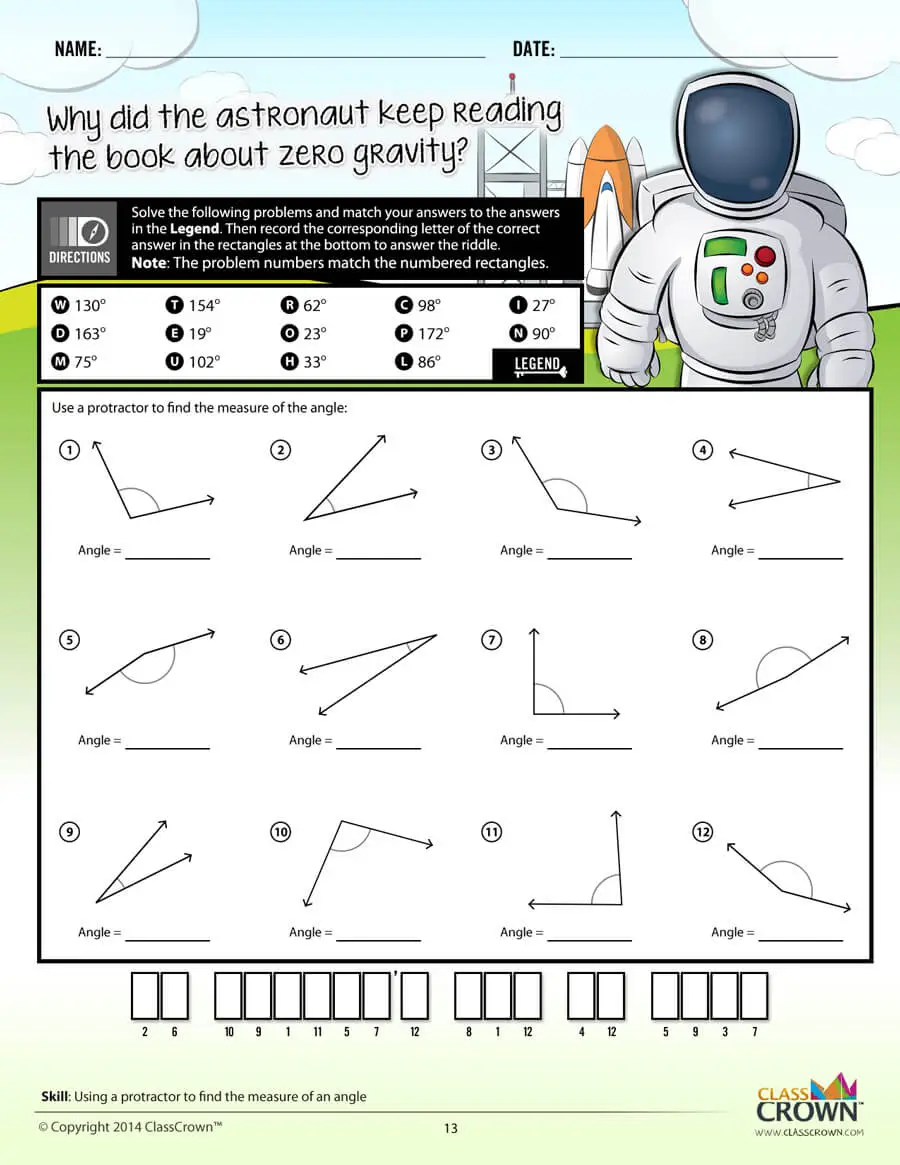Common Core Aligned

All our math worksheet packs are designed with Common Core in mind. That way you don’t have to worry about whether your math ciriculum is aligned or not when you incorpoate ClassCrown Riddle-Me-Worksheets in your lesson plans.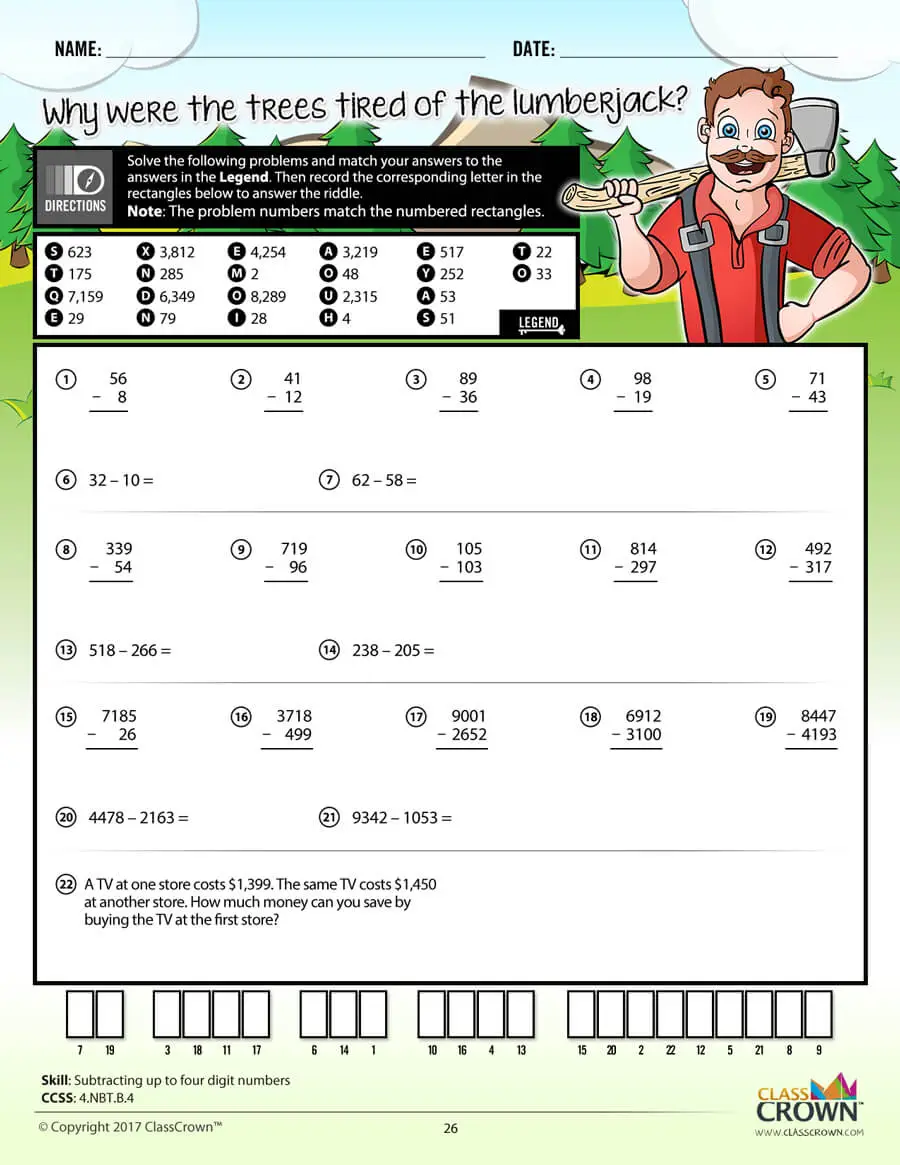High Quality Design

Each page of our math worksheets has been produced in high resolution at 144 dpi and designed in full, vibrant color for maximum quality. They look stunning whether you are printing in color or black and white.High Resolution
(144 dpi)Stunning Color & Clarity